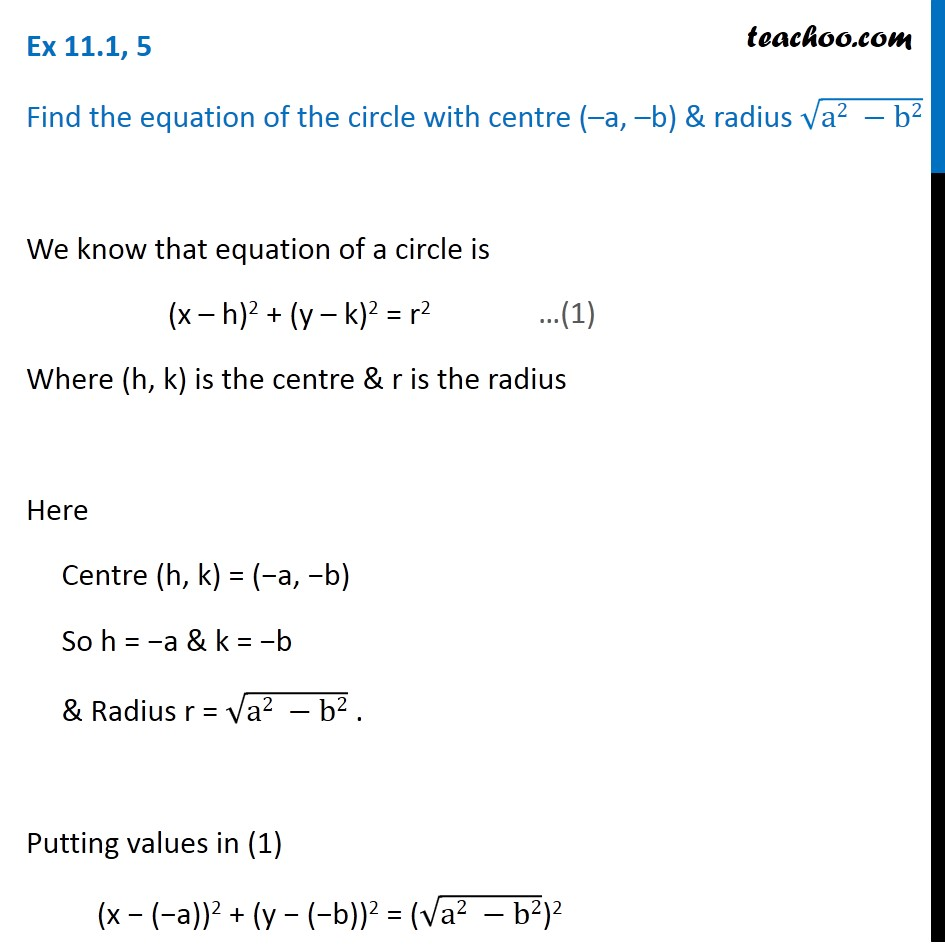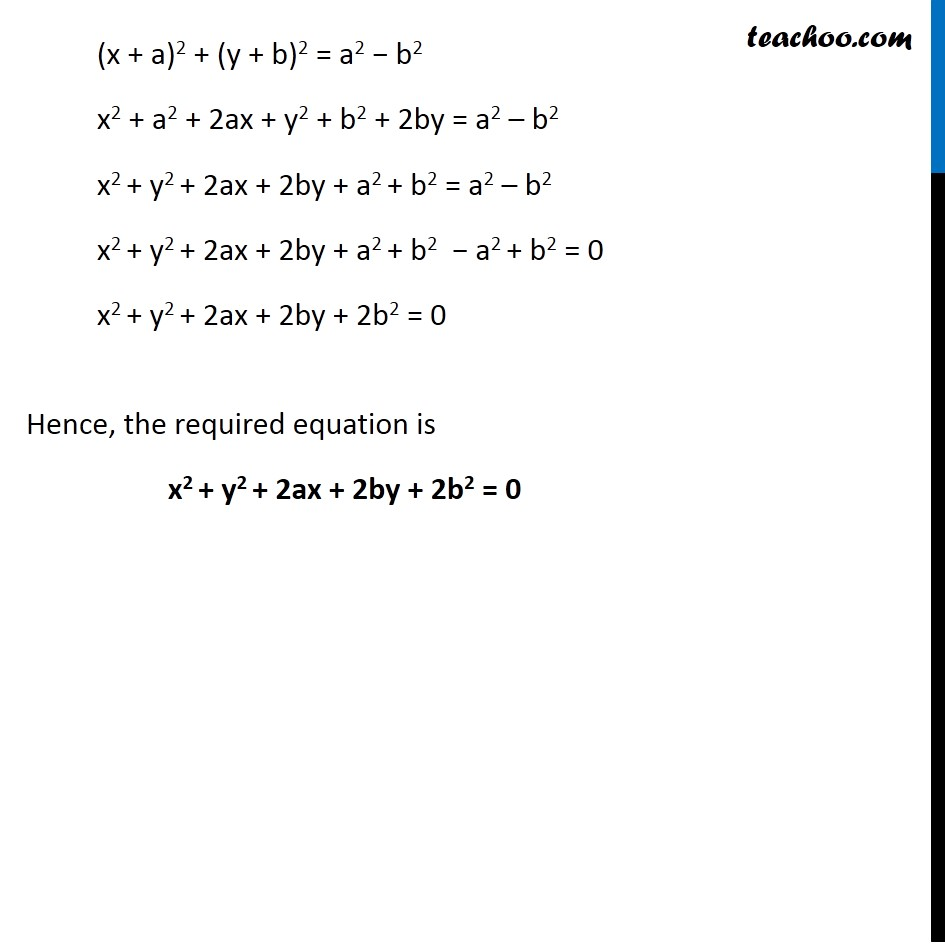Circle

Chapter 11 Class 11 Conic Sections
Concept wiseIntroducing your new favourite teacher - Teachoo Black, at only ₹83 per month

### Transcript

Ex 11.1, 5 Find the equation of the circle with centre (–a, –b) & radius √(a2 −b2) We know that equation of a circle is (x­ – h)2 + (y ­– k)2 = r2 Where (h, k) is the centre & r is the radius Here Centre (h, k) = (−a, −b) So h = −a & k = −b & Radius r = √(a2 −b2) . Putting values in (1) (x − (−a))2 + (y − (−b))2 = (√(a2 −b2))2 (x + a)2 + (y + b)2 = a2 − b2 x2 + a2 + 2ax + y2 + b2 + 2by = a2 – b2 x2 + y2 + 2ax + 2by + a2 + b2 = a2 – b2 x2 + y2 + 2ax + 2by + a2 + b2 − a2 + b2 = 0 x2 + y2 + 2ax + 2by + 2b2 = 0 Hence, the required equation is x2 + y2 + 2ax + 2by + 2b2 = 0